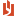## MS Mod 14: Two-factor ANOVA, interaction effects homework assignment

 Author Message NEASSupreme BeingGroup: Administrators Posts: 4.2K, Visits: 1.2K MS Module 14: Two-factor ANOVA, interaction effects homework assignment (The attached PDF file has better formatting.)Homework assignment: Solve either one of the following end of chapter problems, showing all work. The numerical answers are in the appendix to the textbook; for the homework assignment, show how the answer is derived.●    Problem 49 on page 593 (1st ed) / page 606 (2nd ed).    ○    Part (a): The problem gives SSA, SSB, SSE, and SST. Use the appropriate degrees of freedom for each to construct the ANOVA table.    ○    Part (b) tests if any interaction effect is significant at a 5% level. Show the F test and the p value.    ○    Part (c) tests factor A; part (d) tests factor B. Show the F test and the p value.    ○    Part (d) uses Tukey’s honestly statistical difference for factor A. Derive w and Q.●    Problem 53 on page 594 (1st ed) / page 607 (2nd ed).    ○    The problem gives the observed values, not the summary statistics. The totals in the last column and the last row simplify the arithmetic somewhat. Show how to construct the ANOVA table.    ○    Test the interaction effects and the two major effects, showing the F test and the p value. See problem 49 parts (b), (c), and (d) for the items you should address.    ○    Explain why you would not use Tukey’s procedure, given the results of the ANOVA. With a 4 × 3 table and 24 observations of similar size, significant differences are unlikely. AttachmentsMS Module 14 Two-factor ANOVA, interaction effects homework assignment.pdf (141 views, 34.00 KB)
##### Merge Selected
Merge into selected topic...

Merge into merge target...

Merge into a specific topic ID...